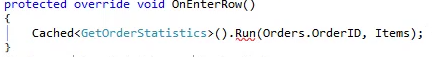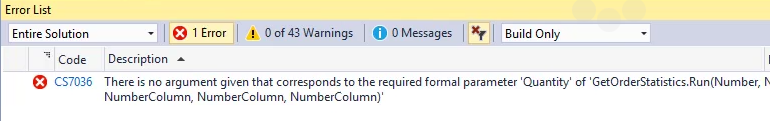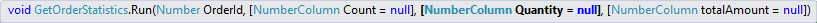﻿ Optional Parameters

# Optional Parameters

• By default, any parameter is a mandatory parameter in C#.
• If we don't send a value into a parameter, Visual Studio will give us a Build error.
• This is the message we'll get when we don't send enough parameters:• To make a parameter optional, in it's definition instead of saying `(NumberColumn Count)` we say `(NumberColumn Count = null)`
``````public void Run(Number OrderId,NumberColumn Count = null,NumberColumn Quantity = null, NumberColumn totalAmount = null)
{
Where.Clear();
_count = 0;
_quantity = 0;
_totalAmount = 0;
Execute();
if (Count != null)
Count.Value = _count;
if (Quantity != null)
Quantity.Value = _quantity;
if (totalAmount != null)
totalAmount.Value = _totalAmount;
} ``````
• Optional parameters are displayed in the parameter information as surrounded by square brakes• If we want to skip an optional parameter, we can use the `null` keyword to skip it when we are calling this method.

Help us improve, Edit this page on GitHub
or email us at info@fireflymigration.com